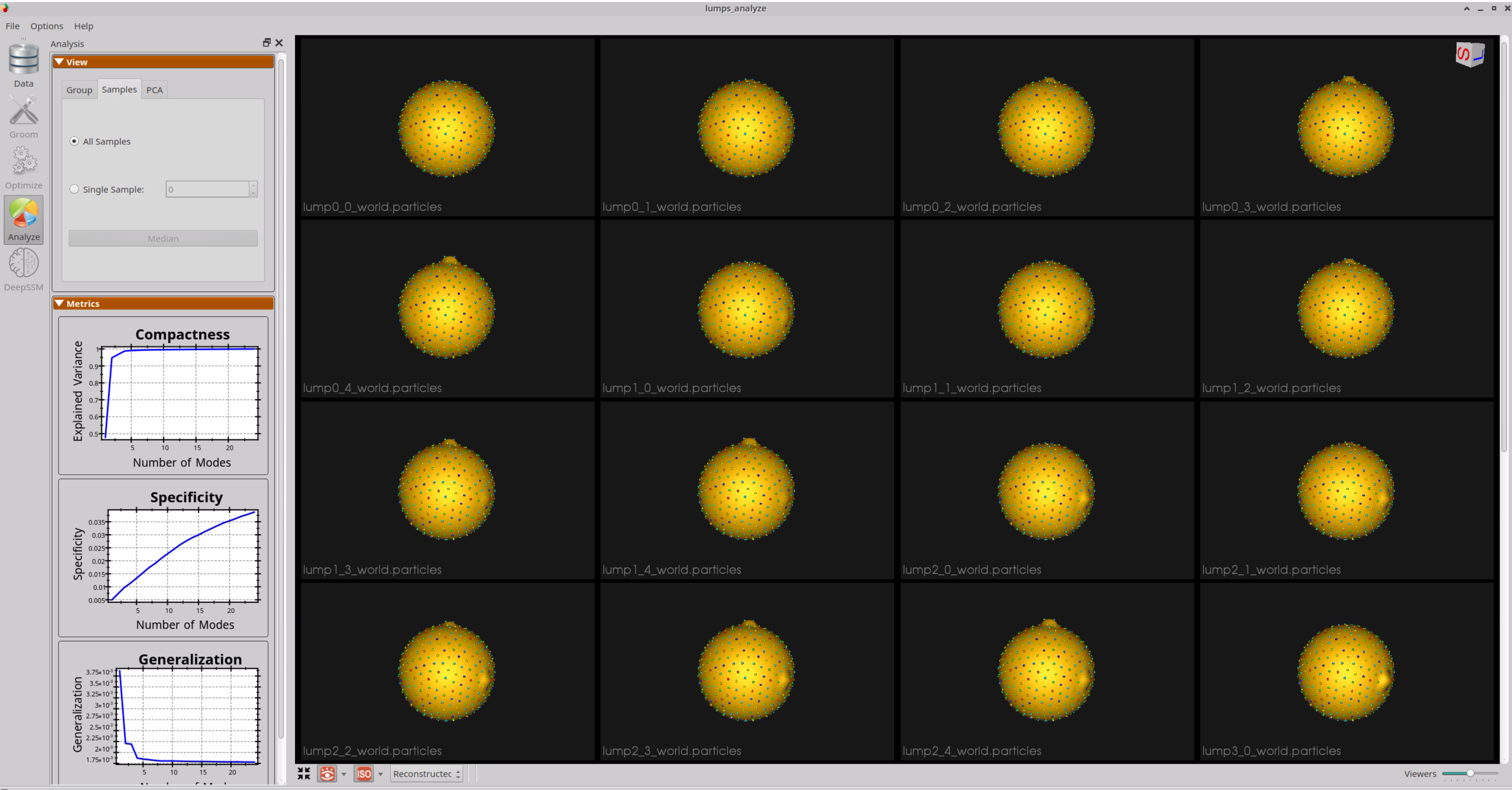# Lumps: Shape Model directly from Mesh

## What and Where is the Use Case?

This use case demonstrates a minimal example to run ShapeWorks directly on a mesh using a synthetic dataset.

The use case is located at: Examples/Python/lumps.py## Running the Use Case

To run the use case, run RunUseCase.py (in Examples/Python/).

$cd /path/to/shapeworks/Examples/Python$ python RunUseCase.py lumps


This calls lumps.py (in Examples/Python/) to perform the following.

• Optimizes particle distribution (i.e., the shape/correspondence model) by calling optimization functions in OptimizeUtils.py (in Examples/Python/). See Optimizing Shape Model for details about algorithmic parameters for optimizing the shape model.
• Launches ShapeWorks Studio to visualize the use case results (i.e., the optimized shape model and the groomed data) by calling functions in AnalyzeUtils.py (in Examples/Python/).

## Grooming Data

This is a synthetic dataset that does not require grooming.

## Optimizing Shape Model

Below are the default optimization parameters when running this use case. For a description of the optimize tool and its algorithmic parameters, see: How to Optimize Your Shape Model.

\$ python RunUseCase.py lumps

{
"number_of_particles": 512,
"use_normals": 0,
"normal_weight": 10.0,
"checkpointing_interval": 100,
"keep_checkpoints": 0,
"iterations_per_split": 2000,
"optimization_iterations": 500,
"starting_regularization": 10,
"ending_regularization": 1,
"recompute_regularization_interval": 1,
"domains_per_shape": 1,
"domain_type": "mesh",
"relative_weighting": 10,
"initial_relative_weighting": 1,
"procrustes_interval": 0,
"procrustes_scaling": 0,
"save_init_splits": 0,
"verbosity": 1
}


## Analyzing Shape Model

ShapeWorks Studio visualizes/analyzes the optimized particle-based shape model by visualizing the mean shape, individual shape samples, and the shape modes of variations. For more information, see: How to Analyze Your Shape Model?.

Here is the mean shape of the optimized shape mode using single-scale optimization. Note the two tiny lumps at the top, and towards the right.Here are lumps samples with their optimized correspondences.Here is a video showing the shape modes of variation (computed using principal component analysis - PCA) of the lumps dataset using single-scale optimization.